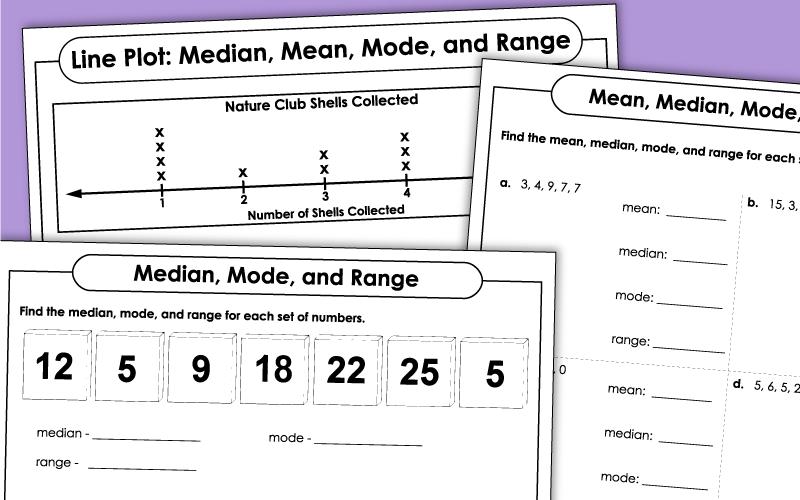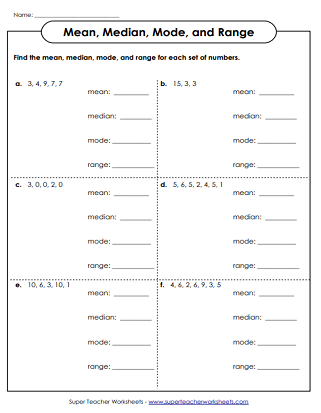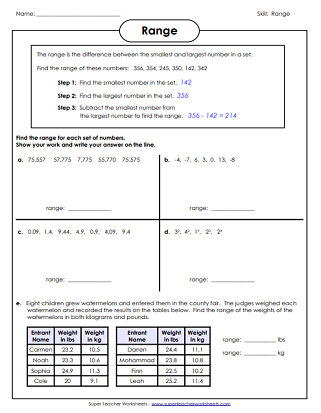# Mean, Median, Mode, and Range Worksheets

Worksheets for calculating the mean (average), median, mode, and range.## Basic Level

Find the median, mode, and range of the numbers shown on the tiles.  This set does not include mean.
Find the mode, median, range, and the mean of the numbers on the tiles.
Use the data on the line plot to answer the range, median, mean, and mode questions.
Find the median of each set of numbers. Includes a word problem.
Find the range for each set of numbers. Includes a word problem.

Find the medians of the sets of numbers. Many sets have two numbers in the middle, and students are required to find the mean of them.
Calculate the range for each set of numbers. Includes negative integers and exponents.

Mean (Averages) Worksheets

These worksheets focus only on calculating the mean, or average.

## Pictures of WorksheetsMy Account
Site Information